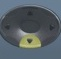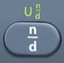## Trapezoid

Trapezoid = Flat Four-Sided Shape with Two Parallel Sides

#Basics
h = height$\bf\displaystyle{{b}_{1}}$ = length of side$\bf\displaystyle{{b}_{1}}$$\bf\displaystyle{{b}_{2}}$ = length of side$\bf\displaystyle{{b}_{2}}$
A = Area = area covering surface of trapezoid

Side$\bf\displaystyle{{b}_{1}}$ and Side$\bf\displaystyle{{b}_{2}}$ are parallel.

Formulas (Provided by Mathematics Formula Sheet)$\bf\displaystyle A=\frac{1}{2}h({{b}_{1}}+{{b}_{2}})$
Parentheses protect order of operations.

Formulas (NOT provided by Mathematics Formula Sheet)$\bf\displaystyle h=\frac{2A}{({{b}_{1}}+{{b}_{2}})}$
Parentheses protect order of operations.

Question
A trapezoidal window is 1200 centimeters tall and its parallel sides are 500 and 800 centimeters long, respectively.  What is its area?

780000 square centimeters$\bf\displaystyle A=\frac{1}{2}h({{b}_{1}}+{{b}_{2}})$$\bf\displaystyle A=\frac{1}{2}$*1200(500 + 800) = 780000

Calculator Click What You See Commentblinker clears screen12× 1200500 + 800$\bf\displaystyle\frac{1}{2}$*1200(500 + 800)$\bf\displaystyle A=\frac{1}{2}h({{b}_{1}}+{{b}_{2}})$780000 Answer
###### Trapezoid

Question
With reference to the question above, what is the area of the window in square meters?

78 square meters

See Measurement.
Square Meters = Square Centimeters ÷ 10000
Square Meters = 780000 ÷ 10000 = 78

Calculator Click What You See Commentblinker clears screen12 × 1200500 + 800$\bf\displaystyle\frac{1}{2}$*1200(500 + 800)$\bf\displaystyle A=\frac{1}{2}h({{b}_{1}}+{{b}_{2}})$780000
780000 ÷ 10000 780000÷10000 Square Meters =
Square Centimeters ÷ 1000078 Answer
###### Trapezoid

Question
A trapezoidal window is$\bf\displaystyle12\frac{1}{2}$ meters tall and its parallel sides are$\bf\displaystyle5\frac{1}{2}$ and$\bf\displaystyle8\frac{1}{4}$ meters long, respectively.  To the nearest tenth of a square meter, what is its area?

85.9 square meters$\bf\displaystyle A=\frac{1}{2}h({{b}_{1}}+{{b}_{2}})$$\bf\displaystyle A=\frac{1}{2}*12\frac{1}{2}(5\frac{1}{2}+8\frac{1}{4})$$\bf\displaystyle\frac{1375}{16}$ = 85.9375 = 85.9 after rounding

Calculator Click What You See Commentblinker clears screen12×1212512+814$\bf\displaystyle\frac{1}{2}*12\frac{1}{2}(5\frac{1}{2}+8\frac{1}{4})$$\bf\displaystyle A=\frac{1}{2}h({{b}_{1}}+{{b}_{2}})$$\bf\displaystyle\frac{1375}{16}$85.9375 Answer
(before rounding)
###### Trapezoid

Question
A trapezoidal window has parallel sides that are 600 and 700 centimeters long, respectively.  Its area is 650000 square centimeters.  What is its height?

1000 centimeters$\bf\displaystyle h=\frac{2A}{({{b}_{1}}+{{b}_{2}})}$$\bf\displaystyle h=\frac{2*650000}{(600+700)}$ = 1000

Calculator Click What You See Commentblinker clears screen2 × 650000500 + 800$\bf\displaystyle\frac{2*650000}{(600+700)}$$\bf\displaystyle h=\frac{2A}{({{b}_{1}}+{{b}_{2}})}$1000 Answer
###### Trapezoid

Practice – Questions

1.  A trapezoidal solar panel is 1500 centimeters tall and its parallel sides are 500 and 700 centimeters long, respectively.  What is its area?

2.  A trapezoidal solar panel is 1400 centimeters tall and its parallel sides are 600 and 900 centimeters long, respectively.  What is its area?

3.  With reference to Question 2, what is the area of the panel in square meters?

4.  A trapezoidal solar panel is$\bf\displaystyle14\frac{1}{2}$ meters tall and its parallel sides are$\bf\displaystyle6\frac{1}{2}$ and$\bf\displaystyle9\frac{1}{4}$ meters long, respectively.  To the nearest tenth of a square meter, what is its area?

5.  A trapezoidal solar panel has parallel sides that are 600 and 900 centimeters long, respectively.  Its area is 900000 square centimeters.  What is its height?